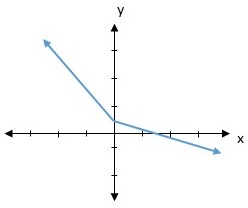# Consider the graph of the function below. Does this function have a critical point at x = 0? ...

## Question:

Consider the graph of the function below. Does this function have a critical point at x = 0?A.Yes.

B.No.

C. There is not enough information.

## Critical Points:

Points on the graph of a function where the derivative is zero or the derivative does not exist are important to consider in many application problems of the derivative. Such points are called critical points.

The given function is a polynomial one, implying it is differentiable in the entire domain. So we just need to find points where the derivative is zero.

We can also tell if the point is a point of maxima or minima by looking at the double derivative of the function.

If {eq}f'(x_1)=0\ \&\ f''(x)>0, {/eq} then the point is point of local minima.

Similarly if {eq}f'(x_2)=0\ \&\ f''(x)<0, {/eq} then the point is point of local maxima.

The above results can be easily obtained after looking at the graph of a general cubic polynomial.

## Answer and Explanation:

Due to the kink in the graph at x=0, we can observe that there is no one unique tangent. Hence derivative of the function at the point doesn't exist

{eq}\Rightarrow {/eq} there is a clear break in differentiability in the function at x=0.

Hence x=0 is a critical point of the given function

#### Learn more about this topic:Finding Critical Points in Calculus: Function & Graph

from CAHSEE Math Exam: Tutoring Solution

Chapter 8 / Lesson 9
163K